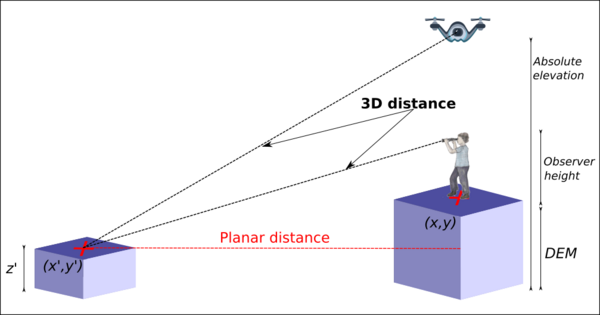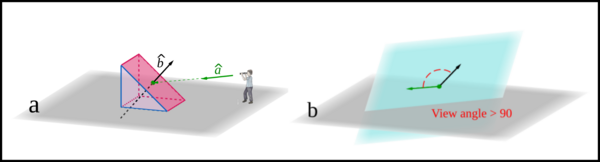## NAME

r.survey - Returns maps of visibility indexes from multiple survey points

## KEYWORDS

raster, visibility, survey, parallel

## SYNOPSIS

r.survey
r.survey --help
r.survey [-bcd] points=name dem=name output=name maxdist=float [treesmap=name] [buildingsmap=name] obs_heigh=float [treesheigh=string] [treesheighdeviation=string] [buildingsheigh=string] [obsabselev=string] [layer=string] viewangle_threshold=float [object_radius=float] [nprocs=integer] [memory=integer] [--overwrite] [--help] [--verbose] [--quiet] [--ui]

### Flags:

-b
Create a simple visible-not visible raster map
-c
Consider the curvature of the earth (current ellipsoid)
-d
allow only downward view direction (used for drone view)
--overwrite
Allow output files to overwrite existing files
--help
Print usage summary
--verbose
Verbose module output
--quiet
Quiet module output
--ui
Force launching GUI dialog

### Parameters:

points=name [required]
Name of input vector map
Name of the input points map (survey location)
dem=name [required]
Name of the input DEM layer
output=name [required]
Prefix for the output visibility layers
maxdist=float [required]
max distance from the input points
Default: 1000
treesmap=name
Name of input vector map
Name of the vector layer representing the forested areas
buildingsmap=name
Name of input vector map
Name of the vector layer representing the buildings
obs_heigh=float [required]
observer_elevation
Default: 1.75
treesheigh=string
field of the table with average threes heigh
treesheighdeviation=string
field of the table with standard deviation value of the heigh
buildingsheigh=string
field of the tablewith buildings heigh
obsabselev=string
field of the table with observer absolute elevation
layer=string
layer of the table of the point map
Default: 1
viewangle_threshold=float [required]
cut the output layers at a given threshold value
Default: 90
radius of the surveyed object (default 0.5 * DEM resolution)
nprocs=integer
Number of processes
Default: 1
memory=integer
Amount of memory to use in MB (for r.viewshed analysis)
Default: 500

## DESCRIPTION

r.survey is aimed at earth surface analysis. It is useful for evaluating the visibility (in terms of 3D Distance, Solid Angle and orientation of the terrain respect to the line of sight) of features lying on the terrain slope, like landslides, road cuts, minor vegetation, mining activity, geological outcrops, surface pipelines, parking areas, solar panels plants, burned areas, etc.

Depending on the purpose of the study, one can be interested in searching the closest position to observe a given territory while for others, having a frontal view can be more relevant. In some cases, the objective could be the combined balance between the closest and the most frontal view, in order to evaluate how much of the human field of view is occupied by a given object (solid angle).

r.survey is deeply based on the powerful r.viewshed GRASS GIS module.

r.survey wants to provide the user the necessary information to answer questions such as:

• In a survey trip, from how many places is something visible?
• During a field work, which place would be the best position to observe a given point/area (according to the distance, the angle or both)?
• Which portion of the territory is visible along a survey path/flight?
• Are objects of a given size visible from a survey path/flight?
Three principal outputs (visibility indexes) are given, based on trigonometric calculations in order to provide qualitative and quantitative information about how the terrain is perceived from the selected observation point (or viewpoint). This information concerns the three-dimensional distance, the relative orientation (View Angle) and the Solid Angle, of each target pixel with respect to the viewpoint.

A map containing a set of points describing the locations of the observer and a digital elevation model (DEM) are the mandatory inputs for r.survey. It is very importante that all observation points have different categories, i.e. cat values (see the examples below). In addition, maps of buildings and trees, portraying height information, can be used to alter the DEM. In the case of trees map, apart from the height value, a third column in the attribute table has to contain the standard deviation value related to the heigh. This is used to simulate the rougness of forested areas.

Many other options can be set according to the aim of the analysis, such as: observer height respect to the ground or respect to an elevation datum (absolute elevation), maximum distance to perform the calculations, a view-angle threshold to exclude, a priori, cell oriented almost perpendicularly to the lines of sight, the average size of the observed object. The input points map must be provided to the tool as a vector layer. In case it represents the positions of a UAV, an helicopter or of a satellite, it must include an attribute field containing absolute elevation values with respect to a vertical datum (the same datum used by the input DEM). Observer height (respect to the ground, the default is 1.75 m) and maximum distance of observation (default is 1000 m) are parameters needed by the r.viewshed module. The object radius is used to approximate, with an equivalent circle, the minimum size of an object centered in the cells center and oriented according to the slope and aspect of the cells. Since r.survey produces different types of outputs, a name to be used for the prefix of the output maps is requested.

3D Distance is the three dimensional linear distance between a viewpoint and a target pixel (see the illutratio bellow). The min3dDistance map portrays the value of the minimum three-dimensional distance between each pixel and the closest viewpoint, in meters.Figure: 3D distance

Given a viewpoint, r.survey calculate the unit vector describing direction and sense of the line of sight between that viewpoint and each visible pixel (see illustration bellow). We define View Angle as the angle between the unit vector representing the line of sight and the unit vector normal to the terrain surface in each pixel. The maxViewAngle map shows the value of the maximum View Angle between each pixel and the viewpoints, in degrees. It is a measure of the most frontal view each single cell is visible from. View angle output is always larger than 90° and smaller than 180°.Figure: View angle

Solid Angle is one of the best and most objective indicators for quantifying the visibility. The idea is that any observed object will be progressively less appreciable the more far away and the more tilted it is with regard to the viewpoint. As a consequence Solid Angle depends on the size of the observed object as well as on the distance and the orientation from where this object is observed. Solid Angle of a surface is, by definition, equal to the projected spherical surface in the evolving sphere divided by the square of the radius of the sphere. As an aproximation, we use the ellipse surface area in place of the projected spherical surface in the evolving sphere. The maxSolidAngle map shows the value of the maximum Solid Angle (expressed in steradians) among those measured from the different viewpoints. In this map, the closest pixels to the observation points have to be interpreted with special attention, considering that the error, respect to the real Solid Angle, can reach until the 10% in the immediate neighbor pixels. For reference: 1 sr is equal to approximately 3283 (deg2) square degrees and to 1.18 x 107 square minutes (min2). It means that 2 x 10-6 sr = 23.6 min2. For comparison, letter acuity (the capacity to resolve a letter) is approximately 25 min2 for a human with perfect vision and in controlled conditions, that is, high contrast between the letters and the background.

Other three maps (pointOfViewWithMin3dDistance, pointOfViewWithMmaxAngle and pointOfViewWithMmaxSolidAngle) are used to register, in each cell, the identifier of the viewpoints from where an observer can get, respectively, (i) the minimum values of 3D Distance, (ii) the maximum values of View Angle and (iii) the maximum value of the Solid Angle. Another relevant output is the numberOfViews map which portraits the number of viewpoints from where each pixel is visible.

## NOTES

The software was designed as a GRASS GIS python module whose source code was written using the GRASS Scripting library and the pyGRASS library.

Multi-core processing is used by r.survey for reducing the computational time. When the aim is to derive the values of the visibility indexes along a given path (e.g. a road or a UAV track) viewpoints can be very dense in terms of number per unit of distance and the more the viewpoints are, the longer the computational time becomes.

Parallel computation was implemented exploiting the Python Multiprocessing library and the ability of GRASS GIS to set a temporary spatial region centered on the considered point without affecting the parallel computation of the other points.

## EXAMPLES

The location used for the following examples can be downloaded here. The name is R.SURVEY_Location

The most common usage of this tool is to model the visibility from the roads, for which a set of points located along the roads is needed. We are going to create a sample of points each 50 meters along the road.
```g.region raster=dem v.build.polylines input=roads output=roads_poly cats=multi  # Convert the road layer into polyline
v.to.points input=roads_poly output=points_50 dmax=50  # A point is created each 50 meters

## Ensure that each point has an independent category value ##
v.category input=points_50 output=points_50_del option=del cat=-1
```

### Running r.survey in parallel

This example shows how to run r.survey calculating the solid angle for an object of radius equal to 20 m and using 4 parallel processes. The height of a tower is considered as well into the calculations.
```g.region raster=Synthetic_valley
r.survey points=Two_viewpoints dem=Synthetic_valley output=example maxdist=3000 buildingsmap=Tower buildingsheigh=Altitude object_radius=20 nprocs=4
```

### Filter output by a view angle threshold

This example shows how to run r.survey calculating the same layers as in example 1, but this time the outputs are filtered by a View Angle threshold of 100 degrees.
```g.region raster=Synthetic_valley
r.survey points=Two_viewpoints dem=Synthetic_valley output=example2 maxdist=3000 buildingsmap=Tower buildingsheigh=Altitude object_radius=20 nprocs=4 viewangle_threshold=100
```

### Using r.survey in flight mode and with the downward view

This example shows how to run r.survey in flight mode and with the downward view. The observation points correspond for the path of an UAV flying in a irregular height above the ground. A field with the points altitude information (above de sea level) is mandatory.
```g.region raster=Synthetic_valley
r.survey -d points=Flight_viewpoints dem=Synthetic_valley output=example3 maxdist=3000 nprocs=4 obsabselev=Elevation
```

## REFERENCES

r.viewshed, r.viewshed.cva, r.viewshed.exposure

## AUTHORS

Ivan Marchesini - Research Institute for Geo-Hydrological Protection (IRPI) - Italian National Research Council (CNR), Perugia, Italy. E-mail: ivan.marchesini@irpi.cnr.it. ORCiD: 0000-0002-8342-3134
Txomin Bornaetxea - Department of Geology, University of the Basque Country (UPV/EHU), Leioa, Spain. E-mail: txomin.bornaetxea@ehu.eus. ORCiD: 0000-0002-1540-3991

## SOURCE CODE

Available at: r.survey source code (history)

Latest change: Monday Jan 30 19:52:26 2023 in commit: cac8d9d848299297977d1315b7e90cc3f7698730

© 2003-2023 GRASS Development Team, GRASS GIS 8.3.1dev Reference Manual